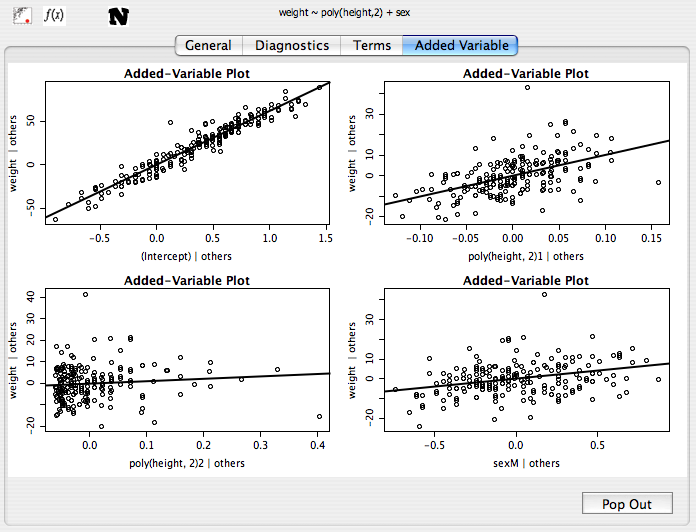## Description

The linear model (also called linear regression) is a flexible framework to analyze the relationship between two or more variables. Linear regression uses one or more independent variables to predict the values of a single continuous outcome variable. The simplest linear model is represented by drawing a best fit line through a scatterplot.

## Variable Selection

This dialog is used to define what variables are of interest, and how they should be treated.

1. Outcome Variable: The dependent variable should be put in this list
2. As Numeric: Independent variables that should be treated as covariates should be put in this list. Note that if a factor is put in this list it is converted to a numeric variable using the `as.numeric` function, so make sure that the order of the factor levels is correct.
3. As Factor: Independent variables that are categorical in nature should be put here (e.g. race or eye color).
4. Weights: This advanced option allows for sampling weights to be applied to the regression model.
5. Subset: As with many other dialogs in Deducer, you can specify that the analysis is only to be done within a subset of the whole data set.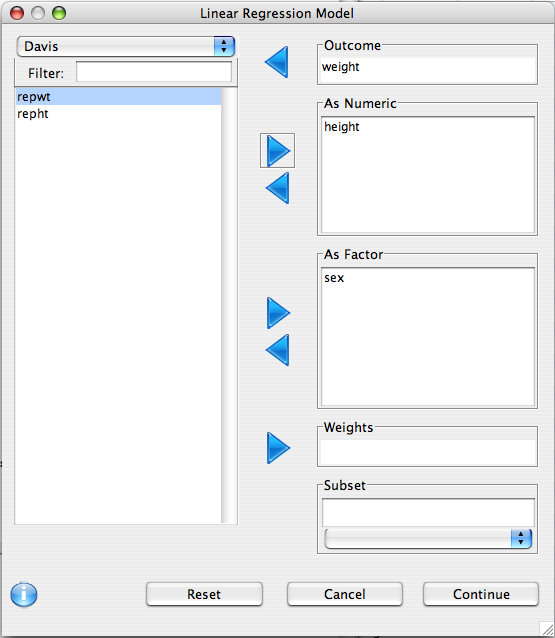## Model Builder

R has a rich syntax for expressing model formulae. The model builder dialog assists in the specification of the terms of a linear regression model.

### Outcomes

Only one outcome is allowed. It can be edited by double clicking on it. for example if we wanted to log transform `weight` for the analysis, we could change it to `log(weight)`.

### Model

Select one or more variables from the Variable list, the click on one of the center buttons to add a term to the model.

• 2-way Add all two way and lower interactions between the selected variables.
• 3-way Add all three way and lower interactions between the selected variables.
• + Add Main effects for all selected variables.
• : Add the interaction between the selected variables to the model.
• * Add the interaction between the selected terms, as well as any lower order interactions between them.
• - Remove a term from the model.
• in Add a nested term to the model
• poly Add orthogonal polynomial terms to the model. A dialog will prompt for the order of the polynomial. If the order is two, this indicates a linear and quadratic term. If it is three it will also have a cubic term. Polynomials can be used when there is non-linearity detected between the outcome and a predictor.

Additionally, terms can be hand edited by double clicking on them.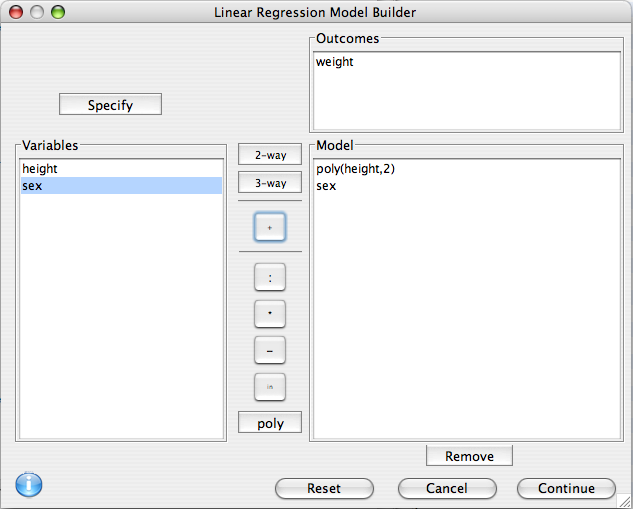## Model Explorer

After the model has been built, its features can be explored. The preview panel displays a preview of what will be displayed in the console when the model is run. In the upper left hand portion of the dialog there are icons representing the assumptions that are being made by the model.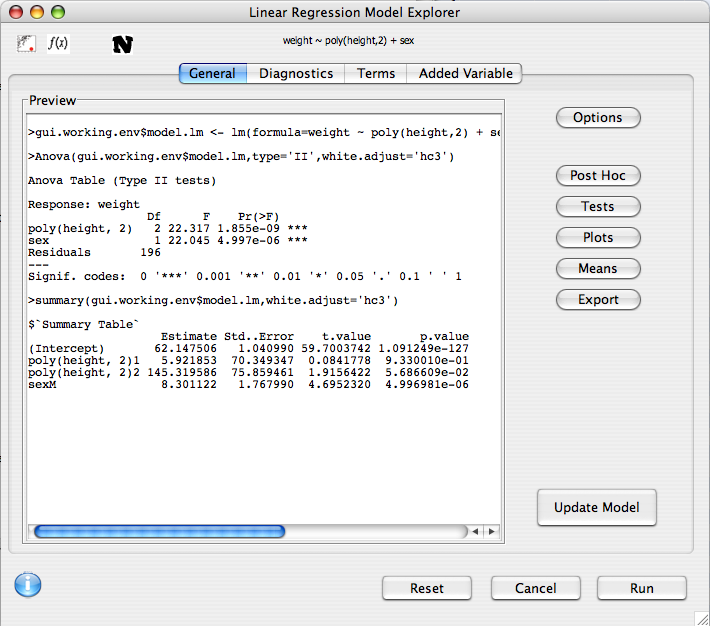The following analysis options and dialogs are available

Additionally, several plots can be accessed through the upper tabs to help diagnose the fit of the model.

### Diagnostics

The diagnostics panel contains 6 plots evaluating outliers, influence, and equality of variance.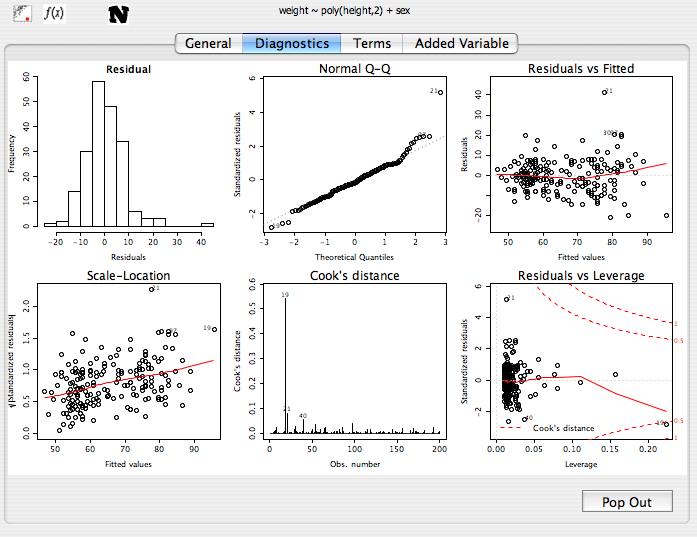• The first two plots describe the distribution of the residuals. Ideally these should be roughly normal.
• Residuals vs. Fitted Shows the residuals of the model plotted against the predicted values. If the red line is not roughly flat, then the model may break down for certain subsets of the data, or there may be significant non-linearity present in the relationships between the predictor variables and the outcome.
• Scale-Location (aka Spread vs. Level) Plots the prediced values vs the square root of the standardized residuals. This investigates the model for departures from equality of variance (homoskedasticity). If there is no heteroskedasticity, the red line should be roughly horizontal. By default Deducer uses methods robust to heteroskadasticity, so there is no need to worry too much about this plot unless the robust option is deselected in the Options dialog.
• Cook's distance Outliers can unduly influence the results of a linear model. This plot shows the row names for observations with moderate or high cook's distance. If the Cook's value is greater than 1, the observation should be examined.
• Residuals vs. Leverage Another plot to examine influence and outliers.

### Term plots (aka Component residual or partial residual plots)

If the model contains no interactions, component residual plots are given. These are used to assess the linearity of the relationship between predictor variables and the outcome.

• For numeric variables, a scatterplot is produced. If the trend line of this plot shows departure from linearity, consider either transforming the variable, or adding polynomial terms to it.
• For factors, box-plots are given. If a factor has more than two levels, is ordinal, and appears to be linearly increaseing or decreasing, consider going back and adding it to the model as a numeric variable.